# multiverse cosmology

Since it appears the universe is an oscillating ejection from an energetic collision, it has hydrodynamic properties common to water droplets.

## LaPlace's Law

The larger the vessel radius, the larger the wall tension required to withstand a given internal fluid pressure.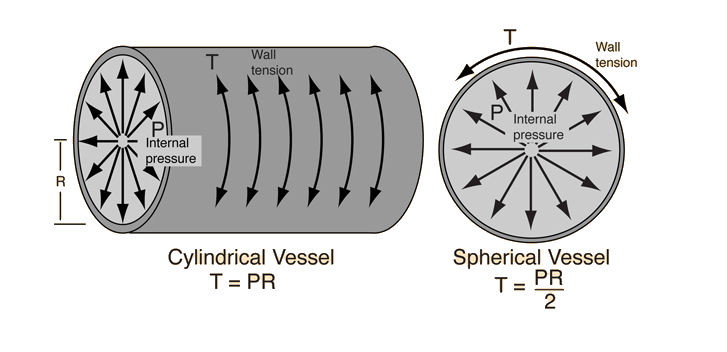For a given vessel radius and internal pressure, a spherical vessel will have half the wall tension of a cylindrical vessel.

In the case of a universe, wall tension is maintained by a density of gravity, which also accounts for accelerated expansion of interior mass (dark energy).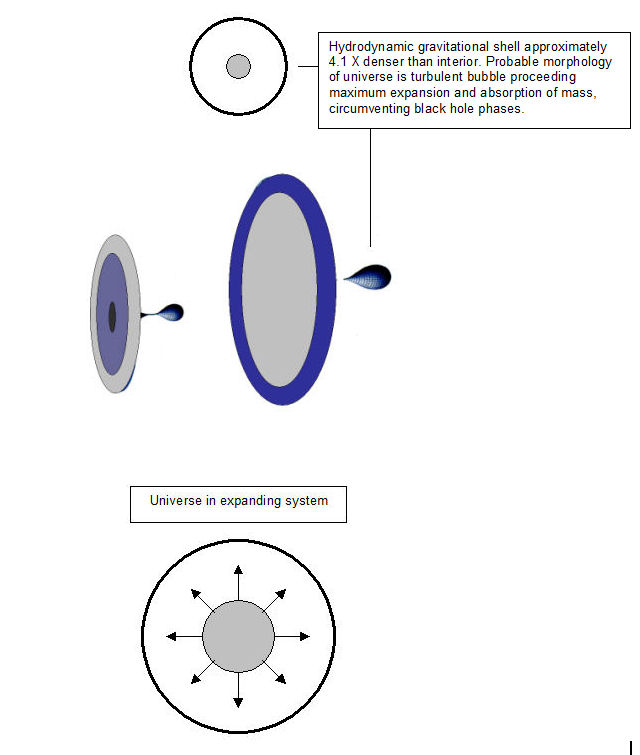As previously noted in cmbr-and-surveys, features are observed in various sky surveys, which indicate large scale oscillation. Gravitational densities (dark matter) are suggested as the spherical domain of the universe and without an oscillating wall density, the features would not evolve.

This source of accelerated expansion constitutes Einstein's cosmological constant as it both attracts interior mass via gravity and contains the universe in a surface tense domain.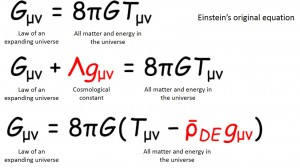Figure 1: Einstein’s equations of general relativity
Gμν on the left side of the equation is the quantity known as Einstein’s tensor, a geometrical description of space-time which, when applied to the universe, describes the extent of its expansion. The right side of the equation is the distribution of all matter and energy contained in the universe. This relationship holds true at every time (instant) in the history of the universe’s expansion. Einstein attempted to produce a steady-state universe solution (static universe) by adding the cosmological constant to the left side of the equation, but when this constant is moved to the right side, it is considered to be a component of energy. In general, including the scenario where the cosmological constant changes over time, this new component is known as dark energy.
© 2014 The University of Tokyo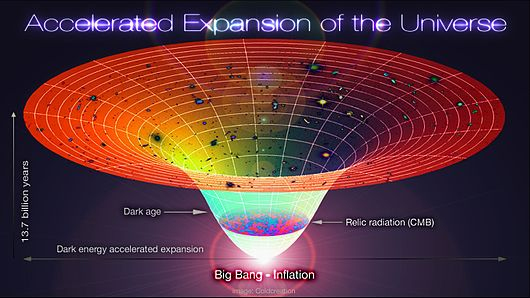Lambda-CDM, accelerated expansion of the universe. The time-line in this schematic diagram extends from the Big Bang/inflation era 13.7 Byr ago to the present cosmological time.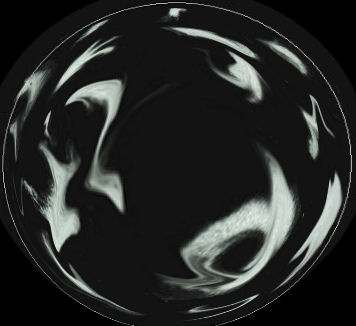Make a free website with Yola